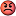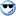A Flash Developer Resource Site

# Thread: Physics again! really lost

1. ## Physics again! really lost

Ok here we go.

A 1900kg car experiences a combinded force of air resistance and friction that has the same magnitude whether the car goes up or down a hill at 27m/s. Going up a hill, the car's engine needs to produce 47 hp (1horsepower=745.7 Watts) more power to sustain the constant velocity than it does going down the same hill. At what angle is the hill inclined above the horizontal?

Any starting tips.Reply With Quote

2. have a look at my diagram first

47hp = 35 047.9W

at a speed of 27m/s this is equivalent to 1298.0703N (X in diagram)

to remain at a constant velocity, all vectors have to be equal (taking g=10m/s^2)

uphill:
N.cos@+(E+1298).sin@ = F.sin@+19000
N.sin@+F.cos@ = (E+1298).cos@

downhill:
N.cos@+F.sin@ = E.sin@+19000
N.sin@+E.cos@ = F.cos@

from these 4 equations you can solve for the 4 variables, N E F @, but i don't feel like doing it for you just yetReply With Quote

3. i'm sure there's gotta be an easier way to do this other than solving for @ - it's proving to be quite a painReply With Quote

4. Well as far as understanding your diagram, I'm on the mark. But as for your equations, I'm lost lol. But thanks for the help, I'm sure once I get my sleep/early morning coffee I'll be able to figure something out.Reply With Quote

5. as there are parallel and perpendicular lines everywhere (add in the horizontal and vertical at the point on the hill) the @ can be moved around with alternate and vertically opposite angles. from there the vectors are resolved into their horizontal and vertical components

to give you an understanding of how this works:

the ascii diagram didnt work so imagine a right triangle with hypotenuse v, base x and vertical side y with angle between hypotenuse and base = @

if the vector is v with angle @ then x and y are the horizontal and vertical components:

cos@ = x/v
x = v.cos@

sin@ = y/v
y = v.sin@

that is what i have done in the earlier explanation and all horizontal components have to cancel in either direction as to vertical components in order to have no accelerationReply With Quote

6. ok so far so good. Ok so, I don't have F, E and @ variables but I have mg which gives me N?

ok so F=E+X
F=mgsin@

so, mgsin@=E+1298. I can't solve for E, I don't have @.

Ok, so N=mgcos@ right. That doesn't make sense, if mg and N=mg, then wouldn't the values cancel out. err.

Ok, I've drawn something on your diagram, to see if I'm looking for the right angle. I used paint so it's a little "butchered" lol. I can see why this question was double starred for difficultyReply With Quote

7. why did you include 19000 in there might I ask? W acts in the vertical, how does that influence the horizontal forces?Reply With Quote

8. Ok is this way possible?
Ok so let me see:

P=FV

P(uphill)=mgsin@ +F(friction)
P(downhill)=mgsin@-F(friction)

ok so I subtract them:

47hp=mgsin@+F - (mgsin@-F(friction)
47hp = 35047 Watts or 1298 N.

1298N=mgsin@-mgsin@+F+F
1298N=2F(friction)
F= 649 N. Funny Friction doesn't cancel out!

hmm,

Cos@=Wx/W
=649N/mg
=649N/18639N
=cos inverse(0.0348)
=88 degrees!

sounds kinda funny, that would be too steep.Reply With Quote

9. you can't just say that N=mgcos@ because there are other vectors working in the vertical as well (parts of E & F)

i included 19000 (equivalent of mg) just to get rid of more variables so it's easier to solve for the others - it is only in the vertical components that it is includedReply With Quote

10. Slosh, help me lol, I'm out of ideasReply With Quote

11. you need to take those 4 equations and solve them simultaneously to get the variables:

uphill:
N.cos@+(E+1298).sin@ = F.sin@+19000 -- (1)
N.sin@+F.cos@ = (E+1298).cos@ -- (2)

downhill:
N.cos@+F.sin@ = E.sin@+19000 -- (3)
N.sin@+E.cos@ = F.cos@ -- (4)

(4)/cos@:
N.tan@+E=F since cos@ doesn't equal 0
N.tan@=F-E

(2)/cos@:
N.tan@+F=E+1298
N.tan@=E+1298-F

therefore:
F-E=E+1298-F (you didnt really need the equations to work that out)

2E+1298=2F
F=E+649

put this into the earlier equation
N.tan@+E=F
N.tan@+E=E+649
N.tan@=649

you repeat this with equation 3:

(3)/sin@:
N.cot@+F=E+19000/sin@
N.cot@=E+19000/sin@-F

F=E+649 (from earlier) so:
N.cot@=19000/sin@-649
N.cos@=19000-649sin@

now you have your two equations for N and @:

N.tan@=649
N.cos@=19000-649sin@Reply With Quote

12. i just realised what an idiot i ami went all the way around with the maths to arrive back at the simplest answer

from the last 2 equations:
N.tan@=649
N.cos@=19000-649sin@

they cancel out to 649 = 19000sin@

this is the exact same as working out the component of gravity that acts along the plane of the hill (mg = 19000)

649 is half of the force difference between uphill and downhill because it acts with the engine and then against it, hence doubling

i could kick myself how easy that is after doing that workReply With Quote

13. wow, that looks complicated lol.

Ok, does this way work?

uphill:-mgsin@-F+(E+1298N)
downhill: -mgsin-E+F

solve for E in bottom and sub into uphill equation.

E=-mgsin@+F

sub into uphill-------> -mgsin@-F+(-mgsin@+F+1298)=0

2mgsin@=1298
mgsin@=649
sin@=649/mg

answer: 1.96 degrees = 2.0 degrees?Reply With Quote

14. BTW, Thanks for the Help!Reply With Quote

15. yup you've got the answer!

i am so annoyed that i never saw that in the first place, you actually don't need to consider any of the other vectors besides mg as mg.sin@ is always the same, down the hill. so you can skip straight to 2mg.sin@=1298N

well at least you now have a mathematical proof of itReply With Quote

####Posting Permissions

• You may not post new threads
• You may not post replies
• You may not post attachments
• You may not edit your posts
•

 » Home » Movies » Tutorials » Submissions » Board » Links » Reviews » Feedback » Gallery » Fonts » The Lounge » Sound Loops » Sound FX » About FK » Sitemap